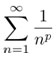# We Use Math Blog

## Millennium Prize Problems

As new discoveries are constantly being made in the field of mathematics, there are still a number of unsolved problems.  Many of these problems, once solved, will help to improve the quality of our daily lives.

In 2000, the Clay Mathematics Institute selected 7 different unsolved problems and offered a prize of \$1 million per problem for those who find a solution.  They chose to call these problems the “millennium problems”. (http://www.claymath.org/millennium/)

These problems include:

• Birch and Swinnerton-Dyer Conjecture
Ever tried to solve a quadratic equation? You just used the quadratic formula right? How about an equation of the form:

y2 = x3 – x

The graph of this equation is called an elliptic curve. It turns out you can describe these curves using algebraic terms and geometric terms. The Birch Swinnerton-Dyer Conjecture says there is a connection between these two descriptions.
http://www.claymath.org/millennium/Birch_and_Swinnerton-Dyer_Conjecture/

• Hodge Conjecture
http://www.claymath.org/millennium/Hodge_Conjecture/

• Navier-Stokes Equations
http://www.claymath.org/millennium/Navier-Stokes_Equations/

• P vs NP
Ever played minesweeper? Ever wondered how fast a computer could beat the game? So do computer scientists! There are very many problems for which it is not known how fast they can be solved. The P vs NP problem seeks to show that these problems can be solved in polynomial time.
http://www.claymath.org/millennium/P_vs_NP/

• Poincaré Conjecture
Solved by Grigori Perelman in the early 21st century.
If something looks like a sphere, smells like a sphere, and tastes like a sphere, is it a sphere? In dimension two, the answer is easy. In dimensions four and greater, the answer is still yes. For a long time, the answer for dimension three was unknown until Perelman solved the problem in the early 2000’s.
http://www.claymath.org/millennium/Poincare_Conjecture/

• Riemann Hypothesis
In calculus you learn that the seriesconverges when p > 1.  People have asked what happens when we treat p as a variable in the complex plane? It turns out this new function is related to prime numbers. The function has zeros at the negative even integers and in a small strip. Reimann’s Hypothesis is that all these extra zeros have real part equal to ½. If this is true, it implies that the primes are well spaced.
http://www.claymath.org/millennium/Riemann_Hypothesis/

• Yang-Mills Theory
http://www.claymath.org/millennium/Yang-Mills_Theory/

## Zero

The number zero was invented independently in India and by the Maya. In India a decimal system was used, like ours, but they used an empty space for zero up to 3rd Century BC. This was confusing for an empty space was also used to separate numbers, and so they invented the dot for a zero. The first evidence for the use of the symbol that we now know as zero stems from the 7th century AD. The Maya invented the number zero for their calendars in the 3rd century AD. The number zero reached European civilisation through the Arabs after 800 AD. The Greek and Roman did not need the number zero for they did their calculations on an abacus.